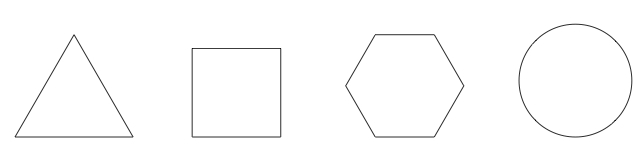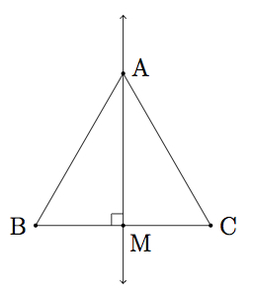# Areas of Geometric Shapes with the Same Perimeter

Alignments to Content Standards: 8.G.B.7

Below are pictures of an equilateral triangle, a square, a regular hexagon, and a circle each having the same perimeter:1. Find the area of the equilateral triangle whose perimeter is $1$ unit.
2. Find the area of the square whose perimeter is $1$ unit.
3. Find the area of the regular hexagon whose perimeter is one unit.
4. Find the area of the circle whose perimeter is $1$ unit.
5. List the answers to (a), (b), (c) and (d) in increasing size. How do you think the area of a regular octagon with perimeter $1$ unit would compare to the triangle, square, hexagon, and circle?

## IM Commentary

This problem is part of a very rich tradition of problems looking to maximize the area enclosed by a shape with fixed perimeter. Only three shapes are considered here because the problem is difficult for more irregular shapes. For example, of all triangles, the one with fixed perimeter $P$ and largest area is the equilateral triangle whose side lengths are all $\frac{P}{3}$ but this is difficult to show because it is not easy to find the area of triangle in terms of the three side lengths (though Heron's formula accomplishes this). Nor is it simple to compare the area of two triangles with equal perimeter without knowing their individual areas. For quadrilaterals, a similar problem arises: showing that of all rectangles with perimeter $P$ the one with the largest area is the square whose side lengths are $\frac{P}{4}$ is a good problem which students should think about. But comparing a square to an irregularly shaped quadrilateral of equal perimeter will be difficult.

For this problem, very explicit shapes have been chosen aiming at providing an opportunity for students to practice using their knowledge of different geometric formulas for area and perimeter. The teacher, in order to reinforce part (d), may wish to draw examples of a hexagon and octagon (both sharing the same perimeter as the other figures). The central idea is that as the number of sides of the polygon increase, the polygon looks more and more like the circle and in particular its area is getting closer to the area of the circle.

Note that the last part of part (e), asking students to think about the regular pentagon, is designed to help them observe that as the number of sides of the regular polygon increases, the polygon looks more and more like a circle. The results of (a), (b), (c), and (d) also might lead to the conjecture that as the number of sides of the polygon (with fixed perimeter) grows, the area increases. While students are not in a position to verify this conjecture, it is good for them to think about the problem. The teacher may also wish to share an interesting historical context of this problem which is described here:

Finally, effort is made in the solution to develop numerical sense in comparing the size of the areas found in parts (a), (b), (c), and (d). If the teacher is interested in stressing this aspect of the task, then it would be appropriate to instruct the students not to use a calculator when doing part (e) of the task. If this aspect is not important, then the answers to (a), (b), (c), and (d) can simply be compared with the aid of a calculator.

## Solution

1. In order to use the formula, Area = $\frac{1}{2} \times$ Base $\times$ Height for a triangle, we need to choose a base and then determine the height of the triangle with this base. Labelling the vertices of the triangle $A$, $B$, and $C$, let $M$ be the point where the perpendicular line from $A$ meets $\overline{BC}$:Note that $|AB| = |AC| = |BC| = \frac{1}{3}$ since the perimeter of triangle $ABC$ is $1$ and all three sides are congruent. Since $\overleftrightarrow{AM}$ is perpendicular to $\overline{BC}$ we can apply the Pythagorean theorem to the right triangles $AMC$ and $AMB$:

\begin{align} |AC|^2 &= |CM|^2 + |AM|^2 \\ |AB|^2 &= |BM|^2 + |AM|^2 \end{align}

We know that $|AC| = |BC| = \frac{1}{3}$ so these two equations show that $|CM| = |BM|$. Since $|BC| = \frac{1}{3}$ this means that both $|AM|$ and $|BM|$ are $\frac{1}{6}$. We now apply the Pythagorean theorem again to triangle $AMC$ to find $|AM|$:

\begin{align} |AM|^2 &= |AC|^2 - |CM|^2 \\ &= \frac{1}{9} - \frac{1}{36} \end{align}

So $|AM|^2 = \frac{3}{36}$ and $$|AM| = \frac{\sqrt{3}}{6}.$$ Now we have found the height of triangle $ABC$, using $\overline{BC}$ as base, and so the area is $$\frac{1}{2}\times \frac{1}{3} \times \frac{\sqrt{3}}{6} = \frac{\sqrt{3}}{36}.$$

2. The square with perimeter one unit has four congruent sides and since their lengths add up to one this means each side is $\frac{1}{4}$ unit in length. The area of the square is length times height so this is $\frac{1}{4} \times \frac{1}{4} = \frac{1}{16}.$
3. A regular hexagon with perimeter $1$ can be divided into $6$ equilateral triangles, each having side length $\frac{1}{6}$ as pictured below.We have found in part (a) the area of an equilateral triangle whose side length was $\frac{1}{3}$ unit. We can repeat the argument from part (a) to find the area of each of these triangles and will find that it will be $\frac{1}{4}$ of the answer from part (a) as the base and height of the equilateral triangle from part (a) have both been scaled by a factor of $\frac{1}{2}$. So the area of the hexagon will be $\frac{6}{4}$ times the area of the equilateral triangle in part (a): $$\text{Area(Hexagon)} = \frac{6}{4} \times \frac{\sqrt{3}}{36} = \frac{\sqrt{3}}{24}.$$

4. The circumference of the circle is $\pi \times$ diameter so if this is $1$ unit then the diameter must be $\frac{1}{\pi}$ units. This means that the radius of the circle is $\frac{1}{2\pi}$ units. The area of the circle is $\pi \times {\rm radius}^2$ so this is $$\pi \times \left( \frac{1}{2\pi} \right)^2.$$ Multiplying this out and simplifying gives $\frac{1}{4\pi}$.
5. Since $\sqrt{3} \lt 2$ we have $$\frac{\sqrt{3}}{36} \lt \frac{2}{36} = \frac{1}{18}.$$ We know $\frac{1}{18} \lt \frac{1}{16}$ so the area of the triangle is less than the area of the square. To compare $\frac{1}{16}$ with $\frac{\sqrt{3}}{24}$ note that if we convert to a denominator of $48$ we have $\frac{1}{16} = \frac{3}{48}$ and $\frac{\sqrt{3}}{24} = \frac{2\sqrt{3}}{48}$. We know that $\sqrt{3} \gt \frac{3}{2}$ as $\left(\frac{3}{2}\right)^2 = \frac{9}{4}$. So $\frac{1}{16} \lt \frac{\sqrt{3}}{24}$. Lastly to compare $\frac{\sqrt{3}}{24}$ to $\frac{1}{4\pi}$ we could use the fact that $\sqrt{3} \lt 1.8$ so $\frac{\sqrt{3}}{24} \lt \frac{1.8}{24} = 0.075.$ On the other hand $4\pi \lt 13$ and so $\frac{1}{4\pi} \gt \frac{1}{13} \gt 0.076$. So $$\frac{\sqrt{3}}{24} \lt 0.075 \lt 0.076 \lt \frac{1}{13} \lt \frac{1}{4\pi}.$$ The triangle with perimeter one unit has the smallest area, followed by the square, then the hexagon and finally the circle. It is reasonable to conjecture, based on this evidence, that as the number of sides of the regular polygon with perimeter one unit increases so does the area. This would mean that the regular octagon would have greater area than the regular hexagon but less than the circle.